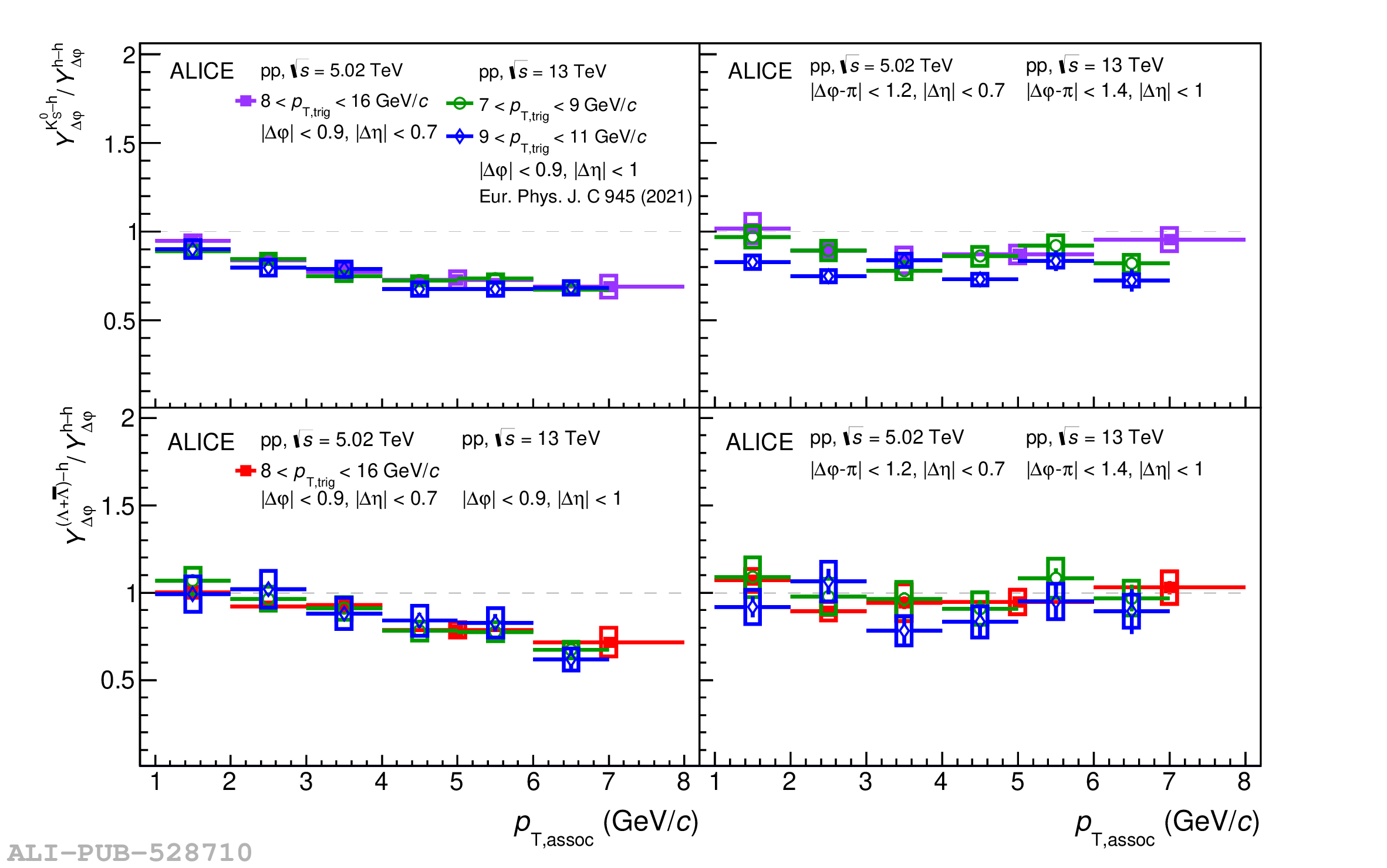# Figure A.1

 Ratios of \Vdecay-triggered yields to charged-hadron (h--h) yields, ${{Y}^{\kzero-\mathrm{h}}_{\Delta\varphi}}/{{Y}^{\mathrm{h-h}}_{\Delta\varphi}}$ (top panels) and ${{Y}^{(\lmb+\almb)-\mathrm{h}}_{\Delta\varphi}}/{{Y}^{\mathrm{h-h}}_{\Delta\varphi}}$ (bottom panels), for \pp collisions on the near side (left) and away side (right) from this measurement compared with published results at higher collision energy .